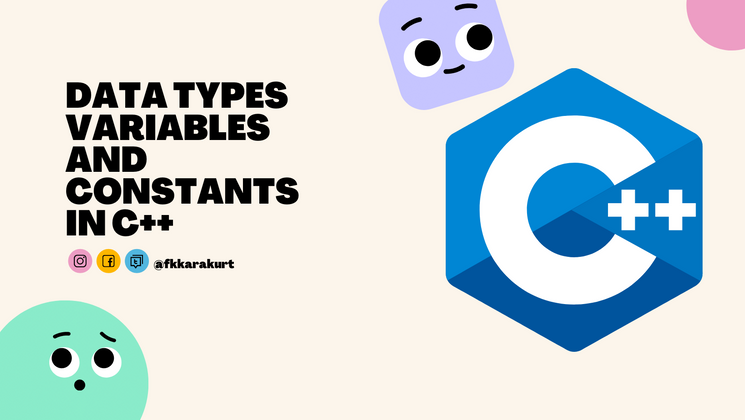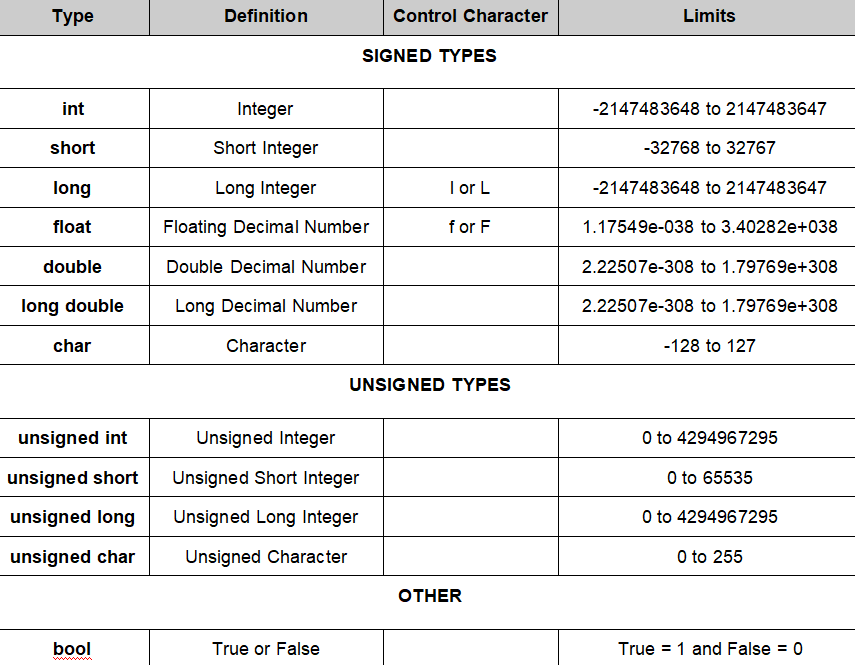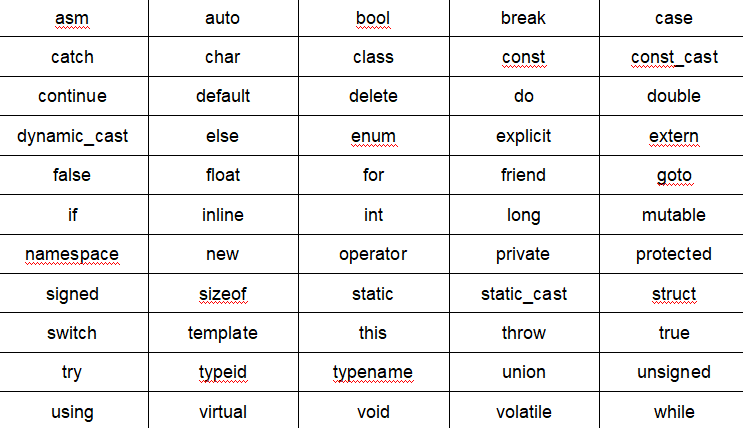## CodeNewbie Community 🌱Fatih Küçükkarakurt

Posted on • Updated on

# Data Types, Variables and Constants in C++

We continue our C++ tutorial series. If you haven't read the introduction, click the link below.

C++ For Beginners

All variables to be used in C++ must be declared and introduced to the program before they are used. During this declaration, the data type of the variable must also be determined.

The basic usage is shown as:

`<datatype> <name of variable>;`

The basic data types commonly used in C++ are:

They are used to define Integers
`<int>` |`<long>`|`<short>`

The `<int>` and `<long>` data types occupy 4 bytes of memory, and the `<short>` data types occupy 2 bytes.

They are used to define decimal numbers

`<double>`|`<long double>`|`<float>`

The `<double>` and `<long double>` data types occupy 8 bytes of memory, while the `<float>` data types occupy 4 bytes.

They are used to identify an alphabetic character or strings of characters

`<char>`

Each character occupies 1 byte of memory.

You can review the table below for the data types used in C++.## Declaration of Variable Data Type

To declare the data type of the variable to be used in C++, a definition is made as follows:

`<datatype> <name of variable>;`

``````int age;
float price;
char letter;
``````

It is possible to change the content of a variable by assigning a specific value anywhere in the program. Often, however, the data type of the variable is determined from the outset, while it is desirable to have a value as well.

`<datatype> <name of variable> = <value>;`

``````int age = 26;
float price = 32.95;
char letter = 'f';
``````

If we are going to use more than one variable in our program, we can define these variables by writing them side by side, provided that they are of the same type.

``````int num1, num2;
int num1=13, num2=14;
``````

Lines where the variable data type is declared, of course, again "`;`" must end with.

Code:

``````#include <iostream>
using namespace std;

int main()
{
int smallest=10;
int largest=100;

cout <<"Smallest Number: " << smallest << "\n";
cout <<"Largest Number: " << largest << "\n";
return 0;
}
``````

Output:

``````Smallest Number: 10
Largest Number: 100
``````

## Variable Naming Conventions

There are some important rules to consider when defining a variable name in C++.

• C++ is case sensitive. e.g;
``````char letter;
char Letter;
char LETTER;
``````

All three of the above statements describe different variables. Therefore, we must be very careful when using lowercase and uppercase letters in variable names.

• No symbols should be used in variable names, except for the numbers, lowercase alphabetic characters, and uppercase alphabetic characters in the table above. However, the underscore (`_`) character is excluded from this scope and can be used in variable names.

• Variable names must begin with a letter or an underscore (`_`) character, never with a number, symbol, or symbol.

``````int num;
int _num;
``````
• The name of a variable can be up to 255 characters.

• Space characters should not be used in variable names. However, the underscore (`_`) character can be used instead of a space.

`int summer_of_sixtynine;`

• C++-specific keywords cannot be used in variable names. These words are given in the table below:## Variables

Variable definitions can be made for different purposes in C++. Although various types of variables are used in C++ programs, for now we will consider 2 types of variables that are frequently used.

### Local Variables:

If there is more than one function in the program, it is the type of variable that can only be valid in the function it is defined in. Such variables must be enclosed in `{ }` signs that indicate function boundaries.

### Global Variables:

It is the variable type that can be valid in all functions in the program. Such variables must be placed outside of the `{ }` signs that specify function boundaries.

### Static Variables:

When a locally defined variable in a function is required to remain constant and not change if the function is called repeatedly as long as the program is running, that variable should be defined as a static variable.

## Constants

Constants are program components whose value does not change from the beginning to the end of the program. Constants with the following data types can be used in C++:

• Integer Constants
• Decimal Constants
• Character Constants
• String Constants

### Integer Constants

There are three types: '`int`' (integer), '`short`' (short integer) and '`long`' (long integer). Let's take 1995 as an example and explain the job of defining the type of an integer in this example.

To indicate which type a constant belongs to, a character is added to the end of that constant to indicate its type. If a numeric expression does not have any characters at the end, the type of that expression is '`int`'. In this case, the expression 1995 in our example is an integer of type '`int`'. To designate this expression as type '`long`' we need to append '`l`' or '`L`' character:

`1995l` or `1995L`. That way the expression now belongs to type '`long`' and not type '`int`' an example and explain the job of defining the type of an integer in this example.

Also, integers that cross the '`int`' type limits in the flow of the program are automatically converted to '`long`', even if they do not have a trailing '`l`' or '`L`' suffix.

There is a special case for the '`short`' type. When calculating the value of an expression, it is treated like '`int`' even though it belongs to type '`short`'. In this case, we can say that there is no constant of type '`short`'. Because constants within the bounds of '`short`' are considered as type '`int`' by C++.

### Floating Constants

There are three types: '`float`' (floating decimal), '`double`' (double decimal) and '`long double`' (long decimal). Let's take the expression 1881.1938 as an example and explain the job of defining the type of an integer in this example.

If a decimal constant does not have any characters at the end, the type of that expression is considered '`double`'. In this case, 1881.1938 in our example is a decimal constant of type '`double`'. To designate this expression as a type 'float' we need to append the '`f`' or '`F`' character: `1881.1938f` or `1881.1938F`. This way the expression no longer belongs to the '`double`' type but of the '`float`' type.

Although not often used, to specify a decimal constant of type 'long double', we must append the character '`l`' or '`L`': `1881.1938l` or `1881.1938L`.

### Character Constants

We know that type '`char`' takes a value between -128 and +127 or 0 and +255. Well, since these constants are named 'characters' and have an alphabetic nature, why are we still talking about numerical expressions?

Because every character used in C++ has a numeric equivalent in the **ASCII (American Standard Code for Information Interchange) **table, and these numbers, in which the character constants are kept within the specified ranges, are the ASCII equivalents of the characters used. In other words; When we talk about 97 as the character constant, we are actually talking about the character '`a`', which is the ASCII table equivalent of 97.

When using character constants, we can use the numeric equivalents of the characters. Of course, the commonly preferred usage in C++ is to use the characters themselves. However, when we are going to use the characters themselves, we must enclose these characters in single quotes (`'`).

In the following lines, two variables of type char are defined and the constants 103 and '`g`' are assigned to these variables, respectively.

``````char character1=103;
char character2='g';
``````

Since the numeric equivalent of the '`g`' character in the ASCII table is 103, these two lines actually mean the same thing. However, pay special attention to the fact that the '`g`' character is written in single quotation marks.

### String Constants

'`string`' (character) literals consist of sequentially ordered strings of character literals. In C++, every expression enclosed in double quotes (`"`) is a constant of type '`string`'. Consider the following examples:

“Fatih" "1995" "1920.1923" "Harvard University"

As you can see, numeric expressions enclosed in double quotes are now a '`string`' constant. We can no longer do numerical operations such as addition and subtraction with them.

Actually there is no type named '`string`' in C++. The '`string`' type occurs when the compiler treats multiple character constants as a string of characters. Accordingly, the expression "`Fatih`" would actually work like this:

`'F' 'a' 't' 'i' 'h'`

The compiler treats all these characters as a string and puts them together by adding the '`\0`' character to the end.

Constants are defined with the word `const` in the C++ program, and the following definitions are made to declare the data type of the invariant to be used:

``````int const constant name = value;
char const constant name = 'value';
``````

Code:

``````#include <iostream>
using namespace std;

int const age = 15;
char const gender = 'M';

int main()
{
cout << " Age: " << age <<"\n";
cout << " Gender: " << gender <<"\n";

return 0;
}
``````

Output:

``````Age: 15
Gender: M
``````

## Type Conversion

Variables or constants in our programs can be of different types. If this is the case, it is important what type of calculation result will be in our mathematical operations. Therefore, type conversion must be done to avoid an error.

Code:

``````#include <iostream>
using namespace std;

int main(){

int num=9;
float a,b,c;

a=num/4;
b=num/4.0;
c=(float)num/4;

cout << "a value= " << a << endl;
cout << "b value= " << b << endl;
cout << "c value= " << c << endl;

return 0;
}
``````

Output:

``````a value= 2
b value= 2.25
c value= 2.25
``````

In the above application:

In the first operation, we divide the variable named `<num>` by an integer value; The decimal point is ignored and the result is assigned to variable `<a>` as an integer.

In the second operation, we divide the variable named `<num>`, which is an integer, by a decimal value; The part after the comma is taken into account and the result is assigned to the variable `<b>` as a decimal value.

In the third operation, we first convert the variable named `<num>`, which is an integer, into a variable of type `<float>`. Next, we divide the variable that is now `<float>` by an integer value; the result is assigned as a decimal value to the variable `<c>`.

## Summary

If you've come this far, congratulations. You are now familiar with the data types, constants, and variables of the C++ programming language. C++ is a language with so many features to explore. So never forget to learn, wonder and research.

It is a difficult language as well as a fun one. But if you enjoy it, C++ will give you more.